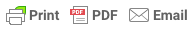Sorry, you do not have permission to ask a question, You must login to ask question. Become VIP Member

Linear measurements in surveying can be performed by mainly three methods namely direct method, electromagnetic methods and optical methods. The direct method is the common method that employs a chain, tape or any other instrument to measure the linear distance.

Also Read: – Electromagnetic Methods in Surveying

Various methods coming under the direct method of linear measurement are:

1. Linear Measurement by Pacing
2. Linear Measurement by Passometer
3. Linear Measurement by Pedometer
4. Linear Measurement by Odometer and speedometer
5. Linear Measurement by Chaining

## 1. Pacing

The pacing technique is mostly employed in preliminary surveys and explorations. In this method, the surveyor is required to conduct a rough survey quickly. This also roughly check the distance measured by other means.

In this method, the number of paces between the two points of the line is counted. Knowing the average length of the pace helps to know the length of the line. The length of the pace is dependent on the person who is measuring, the ground, the speed of pacing and the slope of the country.

By walking on an approximate level ground over various lines of known lengths helps to determine the length of one’s natural step. Performing pacing over rough ground or on slopes is a difficult process.

## 2. Passometer

The passometer is a watch like instrument that is carried on the person’s pocket or tied on the man’s leg. This instrument records the paces when the man moves from one point to another.

This technique hence overcomes the monotony and strain in counting the paces by the surveyor. The distance is calculated as the product of the number of paces and the average length of the pace.& Ad Free!

GET VIP MEMBERSHIP NOW!

## 3. Pedometer

Pedometer is a similar device like passometer that automatically measures the distance. A pedometer is best in measuring the vertical distances.

## 4. Odometer

Odometer is an instrument that measures the number of revolutions of a wheel. The number of revolutions taken to cover the length multiplied by the circumference of the wheel gives the value of the distance covered.

This instrument does not provide accurate results on an undulating surface. When the surface is smooth, the speedometer used in the vehicles can be used.

## 5. Chaining

Chaining method determines the distance by means of a tape or a chain. This is one of the accurate methods to determine the linear measurements. The chain is used for ordinary precision. Tape or a special bar is used to measure distance with high precision.

Also Read: What is Chain Surveying?